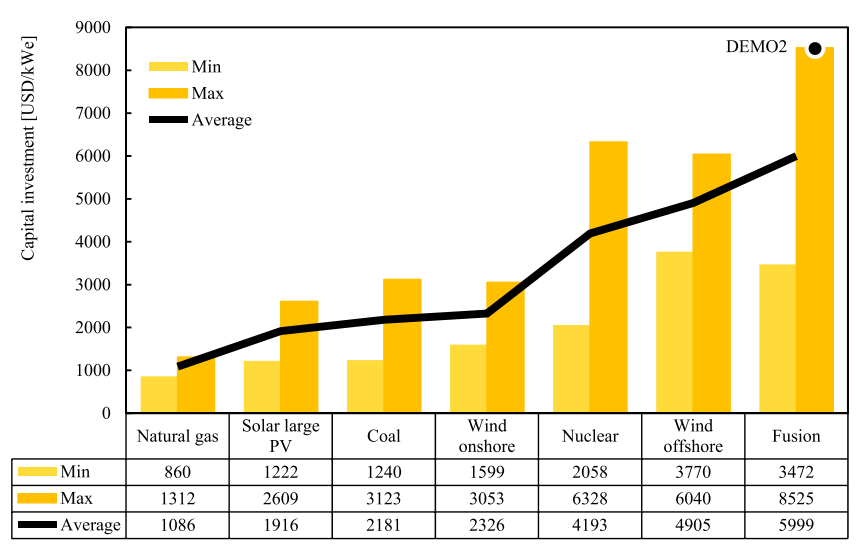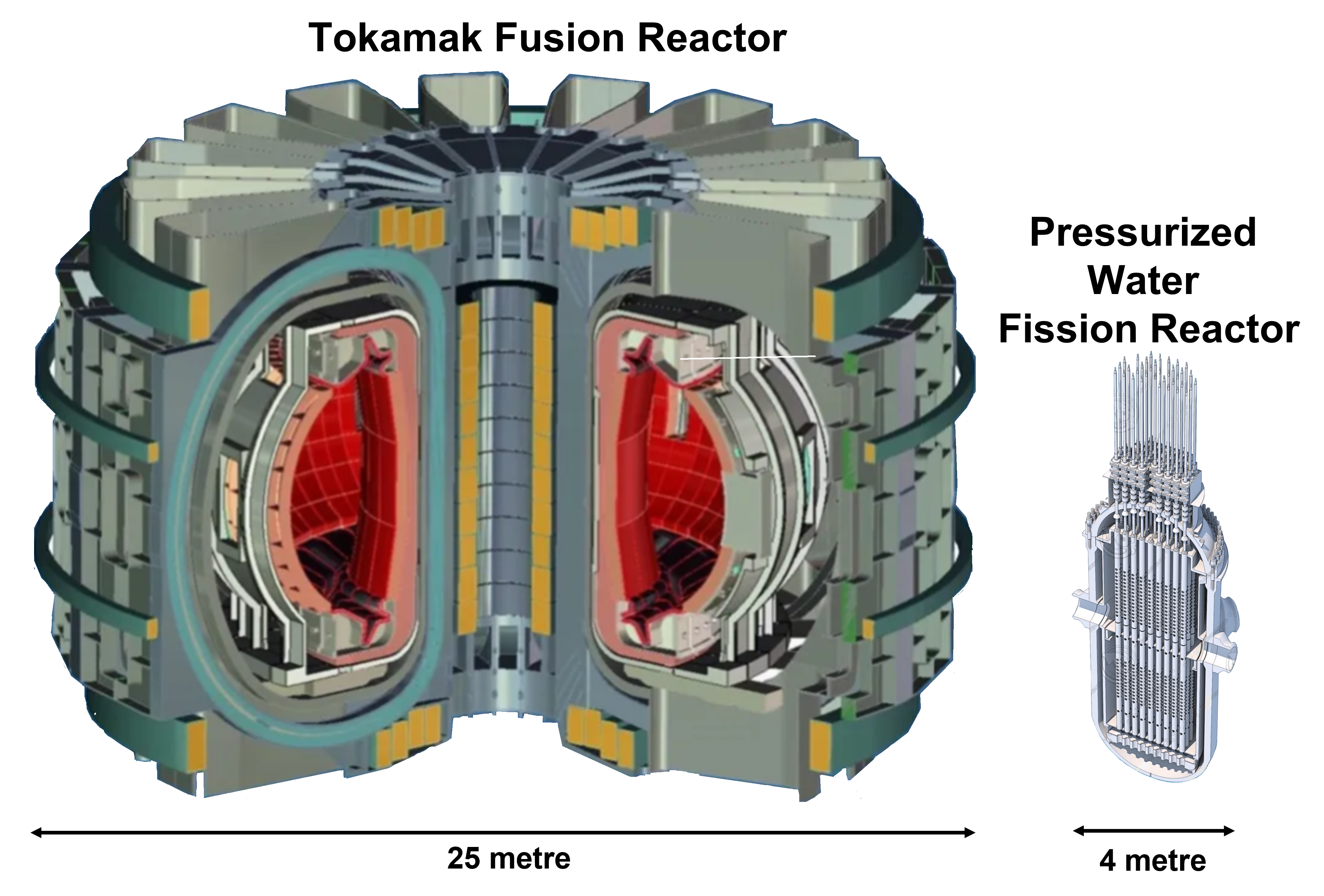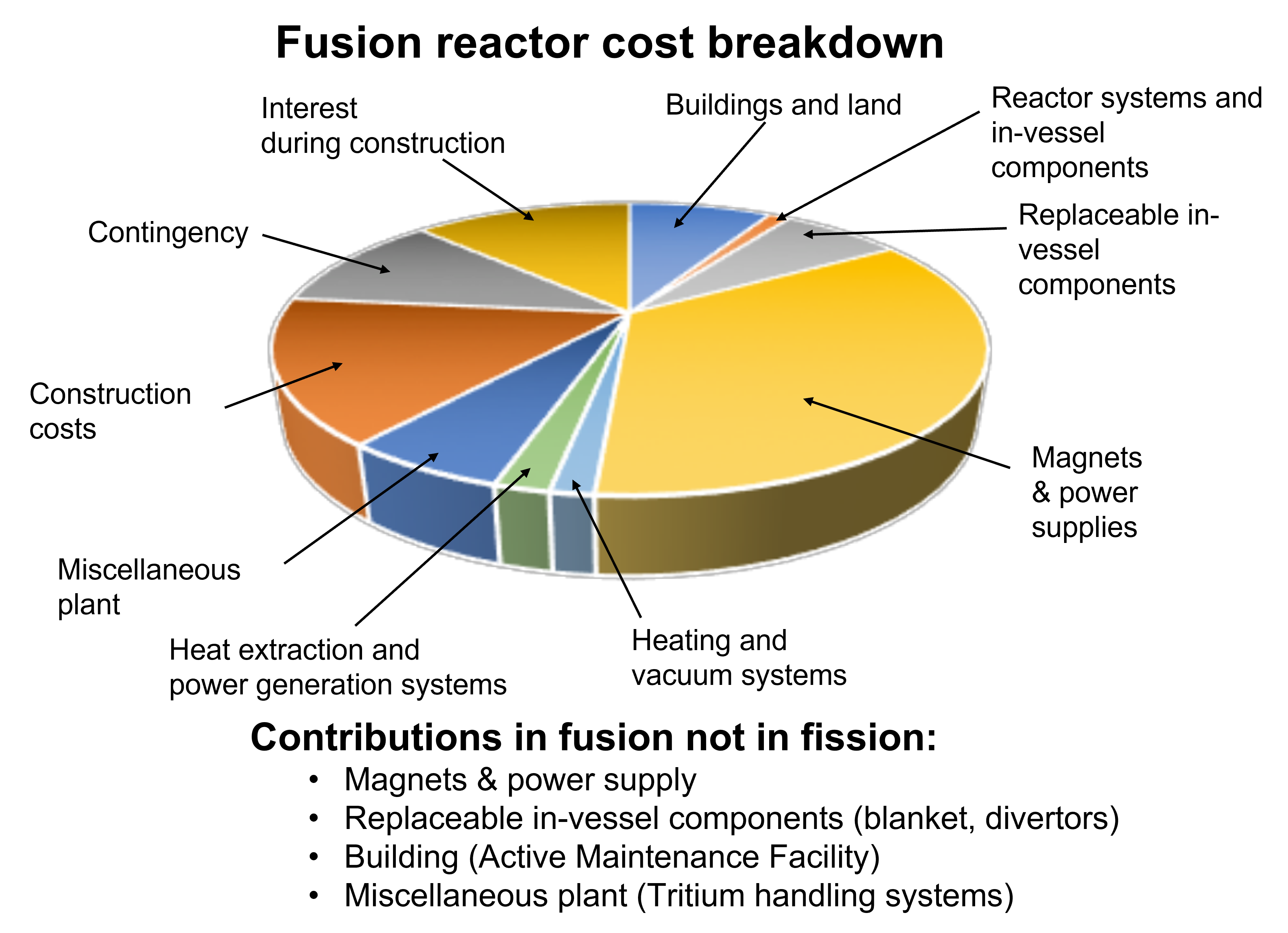Costs are a vital ingredient to evaluate fusion but, unfortunately, are very badly known at the present time. Fusion advocates suggest the electricity price from fusion will be competitive with fission, but it is more likely that the actual cost of electricity from fusion would be several times that of fission. Various estimates are presented on this page.

### Estimates based on ITER construction costs

Some estimates for costs of Fusion Power Plant  have been made based on the ITER construction cost. This is complicated by the fact that the ITER costs include in-kind contributions which are treated differently by the various countries involved, leading to estimates of ITER cost in the range \$22-65 billion. Reinders in his book, The Fairy Tale of Nuclear Fusion, suggests based on ITER, fusion costs would be about 4 times more than fission. Hirsch in an article, Fusion: Ten Times More Expensive Than Nuclear Power, suggests fusion as 10 times more.

### Published fusion reactor cost estimates

The paper by Entler et al.: Approximation of the economy of fusion energy makes an evaluation of the fusion costs, compared to other technologies, and these are illustrated in the following figure. This data gives a range of investment costs for fission between 2058-6328 \$/KW and fusion between 3472-8525 \$/KW. The average of these ranges give fusion about 1.4 times that of fission but the difference between max and min for both fusion and fission are close to a factor of 3, indicating a very large uncertainty on the relative cost.Construction costs of fusion compared to other electricity technologies. The plot (from Entler et al.) gives the maximum and minimum range from various studies.

### Estimate from volume of reactor

Crude estimates for the relative costs of fusion and fission can be obtained by comparing the scale of material and the different systems needed for the reactors. The figure below compares the scale of a fusion reactor and a fission reactor with similar (1 Gigawatt) electrical power output.Indication of relative sizes of a 1-Gigawatt electrical power fusion reactor compared to a fission reactor producing the same power.

A fission reactor is very much smaller than a tokamak. The former has a volume about 100 m3while a Tokamak, with similar power output, would be more than 10000 m3. This indicates that material costs for a fusion reactor would be about a factor 100 more than fusion. This number does not allow any estimate of manufacturing costs but gives the crude deduction that fusion costs a lot more than fission.

### Estimate from extra systems

A better estimate for the construction cost of a mainstream fusion power plant is made by costing the extra systems in a fusion plant compared to a fission plant. This estimate uses the breakdown of projected costs for a fusion power plant from the PROCESS reactor design code, given in a paper by Richard Kembleton with the plot below showing the contributions.Basis for cost estimate using in information in paper by Richard Kembleton.

The cost estimate made here, takes from the Kembleton analysis only component costs and excludes, construction, contingency and interest. Then the comparison is made by identifying the extra items in fusion which do not appear in fission. The contributions indicated beneath the pie chart, are dropped completely for fission. For "Building" and "Miscellaneous Plant" in the pie chart, it is assumed that only half would be in a fission power plant. The items "Heat extraction and power generation systems" as well as "Reactor systems and in-vessel components", are assumed similar for fission and included in the relative fission estimate unchanged. With this, fusion comes out four times more expensive.

### Electricity production capacity factors

For any electricity generation technology, the proportion of time the power plant produces electricity, capacity factor, is vital in the real electricity cost.  Nuclear fission typically has the highest capacity factor of any electricity generation technology (about 90%), while for nuclear fusion this could be only 30%. [Maintenance, Breakdowns and Availability]. This effectively means fusion would be more expensive than fission by a further factor three.

### Cost of operations

Operational costs are important but also difficult to calculate. Again, an attempt at an estimate can be made by comparing to fission. Fuel costs for fusion for start-up tritium will be astronomical because of the near non-existence of supplies, [Lack of tritium fuel]. The multiple auxiliary systems of a tokamak such as cryogenics, independent cooling systems; tritium purification plant and frequent component replacements mean high manpower costs and maintenance material costs. The factor relative to fission for all of this will be at least a factor two.

### Conclusions on Fusion Costs

This page has shown the construction cost of a fusion power plant would much greater than fission. The volume-based argument alone can demonstrate as ridiculous any claim that fusion costs would be similar to fission costs.

The specific estimate made here suggests fusion power would be much more costly than fission with the following contributions.

• Construction costs: a factor 4 more. This is in the middle of the range of the other estimates quoted.
• Capacity factor (availability) a factor 3 less. This is not included in the other, published, estimates.

These estimates can be combined to give a cost of electricity 12 more for fusion than for fission.

• Operational costs a factor 2 more, but this relative factor cannot be added to the overall factor without estimates of absolute costs.

The final conclusion here, is that the costs of a mainstream fusion power plant are unknown at the present time but certainly will be much higher than fission by more than a factor 10.